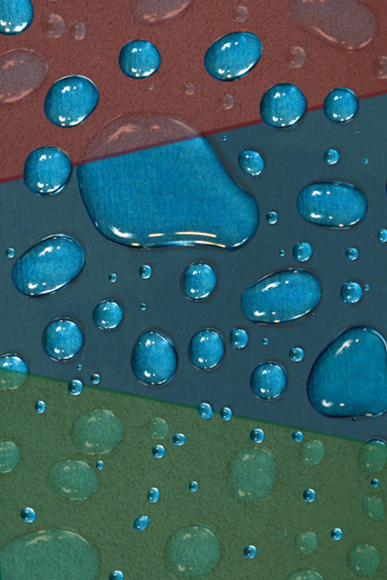﻿ DigitalworksPartitions
 Complex systems having a large number of units or constituents (such as a gas composed of huge number of molecules, etc.) are difficult to handle mathematically if one wants to keep track of each constituent. There are simply too many equations to solve! Physicists have devised a way to deal with this problem. The system is partitioned in a way that each partition contains units with similar properties. In the picture the system contains a large number of water drops of varying sizes. Each partition, shown as green and red surfaces, picks up drops of similar sizes. This trick reduces the complexity and makes the system tractable mathematically.